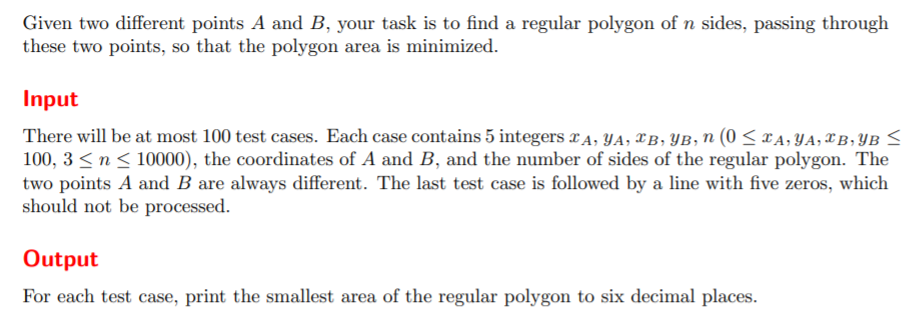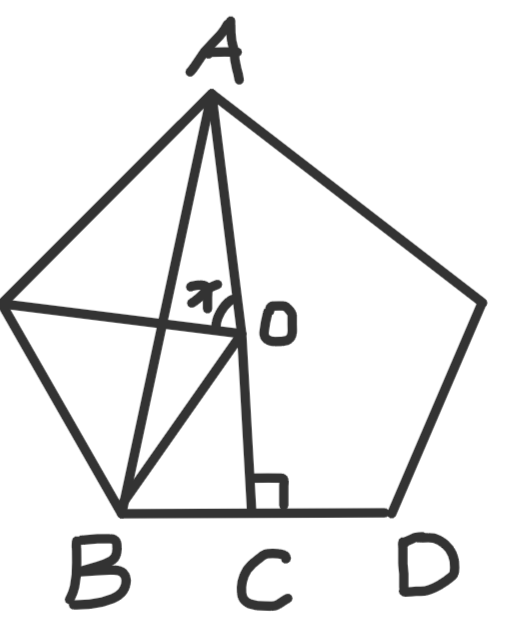# UVA12300 Smallest Regular Polygon(计算几何基础)Sample Input
0 0 1 1 4
1 2 3 4 5
2 3 4 5 6
0 0 0 0 0

Sample Output
1.000000
5.257311
5.196152

• n 为偶数的时候，r 就是这两点之间的距离的一半
• n 为奇数时，设这两点之间的距离为 d，那么半径   ，这是当　ｎ＝５　的时候
• 如下图所示，我们需要计算　∠ＡＯＢ　的一半，所以：
•  再正 n 边形中，AC 垂直于 BD，那么 ∠AOB=pi-x/2
• 所以
•int n,m,t;
struct Point
{
double x,y;
Point(double x=0,double y=0):x(x),y(y){}
};

typedef Point Vector;
Vector operator-(Vector a,Vector b){ return Vector(a.x-b.x,a.y-b.y); }
double Dot(Vector a,Vector b){ return a.x*b.x+a.y*b.y; }
double length(Vector a){ return sqrt(Dot(a,a)); }

int main()
{
//IOS;
Point a,b;
while(~sff(a.x,a.y)){ sff(b.x,b.y); sd(n);
if(n==0) break;
double d=length(a-b);
double r=d/2;
if(n&1){
r=d/2/sin(sita/2);
}
printf("%lf\n",ans);
}
//PAUSE;
return 0;
}

12-1248808-15606
03-28841
02-05530
11-104万+
04-11667
10-22312
06-291901
08-231516
03-25
09-28
04-28
12-0815万+